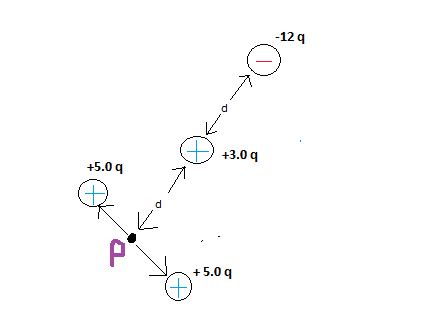# Finding the electric field at point p in this formation?

## Homework Statement

What is the magnitude of the electric field at point p due to the four point charges shown? The distance d is between charge centers.## Homework Equations

Electric Field = kq/d^2
Coulomb's Law = k(q1q2)/d^2

## The Attempt at a Solution

I don't know how to go about solving this for a multiple of reasons. First of all, the formation is really throwing me off. I think both the positive and negative 5 charges cancel out, but I'm not too sure how to calculate the forces on p due to the +3.0 and -12

gneill
Mentor
I don't know how to go about solving this for a multiple of reasons. First of all, the formation is really throwing me off. I think both the positive and negative 5 charges cancel out, but I'm not too sure how to calculate the forces on p due to the +3.0 and -12
Hi shqiptargirl, Welcome to Physics Forums.

I don't see any negative 5q charges, just two positive 5q charges. But you're right about their fields cancelling at P.

Note that you're looking for the field contributions of the other charges at point P, not forces (there's no charge at P on which a force could be developed). Use your electric field equation from your Relevant equations. What are the distances of the +3q and -12q charges from point P?

That's what I have trouble understanding. I know the electric field at point E due to the +3 charge would be (9 * 10^9)(3)/d^2. And the electric field at point p due to the -12 charge would be (9 *10^9)(12)/(2d)^2. But I don't know where to go from there. How do I add them together if the distance remains unknown?

I am also confused because one charge is negative. Do I include the negative symbol when calculating the electric field?

gneill
Mentor
That's what I have trouble understanding. I know the electric field at point E due to the +3 charge would be (9 * 10^9)(3)/d^2. And the electric field at point p due to the -12 charge would be (9 *10^9)(12)/(2d)^2. But I don't know where to go from there. How do I add them together if the distance remains unknown?
Note that you're not given a value for q either. Being of a suspicious nature, I'd look for a trick if you're asked for a numerical result. Add the two field magnitudes up symbolically and see what it looks like.

That's what I don't understand unfortunately. How do I add up the two fields? I know I have to make the denominators equal first. So I would multiply both the denominator and numerator of (9*10^9)(3)/d^2 by 2. From that point on I am confused. Am I suppose to distribute the 2 to both (9*10^9) and to (3) ?

gneill
Mentor
You should forget the numerical value for the Coulomb constant and just write "k" for now, since you're doing a symbolic manipulation.

##E = k \frac{3q}{d^2} + k \frac{-12q}{(2d)^2} ##

See if you can simplify the second term a bit first.

Okay, with this I got k *6q/2d^2+ k*-12q/2d^2. Can I know add them together to get k* -6q/2d^2 and then simplify to get -3q/d^2?

Also, how do you format your fractions so nicely?

gneill
Mentor
Okay, with this I got k *6q/2d^2+ k*-12q/2d^2. Can I know add them together to get k* -6q/2d^2 and then simplify to get -3q/d^2?
Something's gone awry in your algebra. Take note that the (2d) in the denominator of the second term is ALL squared. How do you expand ##(2 d)^2 ##? Cancel what you can there in that term.
Also, how do you format your fractions so nicely?
I'm using Latex syntax. If you hit "Reply" on one of my posts with it in it you'll see the tags and such.

Okay I think I figured it out. If I expanded ##E = k \frac{-12q}{2d^2} ## I'd get ##E = k \frac{-12q}{2d*2d} ## which would simplify to -3?

The thing I don't understand is how I can simplify this fraction when there is a variable D in the denominator. How can I take 2d out of 12 if I don't know what d is?.

And so overall, the net electric field would be 0?

gneill
Mentor
Okay I think I figured it out. If I expanded ##E = k \frac{-12q}{2d^2} ## I'd get ##E = k \frac{-12q}{2d*2d} ## which would simplify to -3?

The thing I don't understand is how I can simplify this fraction when there is a variable D in the denominator. How can I take 2d out of 12 if I don't know what d is?.
You don't simplify further when you have different variables. You just reduce to simplest terms, which in this case for for the expression in question is

## k \frac{-3q}{d^2}##

Lo and behold, that just happens to be the negative of the first term of the sum! A fine (no doubt intentional) coincidence.
And so overall, the net electric field would be 0?
Yes indeed :)

Thank you so much for all your help! I understand it now ;)